## Qubits and Gates - Quantum Computer Programming

Quanum-prog-2-the-qubit

Hello and welcome to part 2 of the Quantum computer programming tutorials. In this tutorial, I want to talk a bit more about qubits, and subsequently their gates. If you can believe it, I only told you part of the story!

Before this, I want to take a moment to address some of the most common questions that I got from part 1.

### Possible states vs considering states

There still seemed to be some confusion about the difference between a classical computer's ability to analyze states and a quantum computer. The thing I heard most was that classical bits can store 2^nbits of information. This is *of course* true. Given n bits, there are 2^n_bits combinations possible. But, can a classical computer actually consider 2^n_bits of possibilities at once? In true parallel? No. This is what a quantum computer *can* do. Given a single pass through a classical circuit, you can only consider 2*n_bits. A quantum computer, on a single pass through a quantum circuit, can consider up to 2^n_bits *logically*, all at once, in parallel. This is the quantum difference. That is what you're going to struggle with mentally to make sense of.

### Back to our Qubit story...

To tell the rest of the story, we will visualize the qubit as a vector in space. The notion that a qubit is a 0, 1, or both is not quite true. The measured qubit will collapse to a 0 or 1 value, yes, and if a qubit starts out with no operations, sure, it's a 0. Then if we apply a not gate, it's going be a 1...but this still isn't the full story of what's happening, or, more importantly, what can happen.

We will use the Bloch sphere to visualize this.

Bloch Sphere

We use the Bloch sphere to represent our Hilbert Space.

A Hilbert space is the extension of a Euclidean space, just for infinite dimensions.

Our qubit is represented by a vector in this space. This vector doesn't necessarily map to a 0 or 1 value

For example, here's our bloch sphere: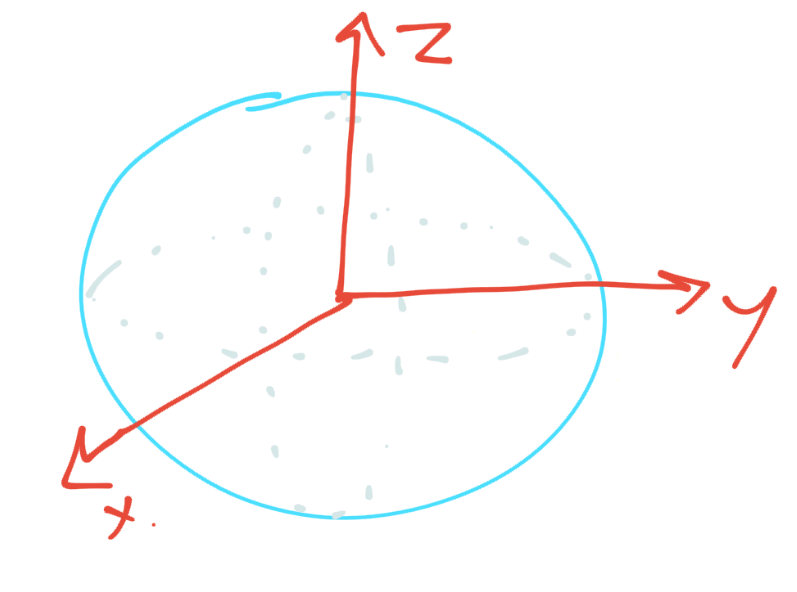Very pretty, I know. This sphere, at the top and bottom, have values that we can map to classical 0 or 1: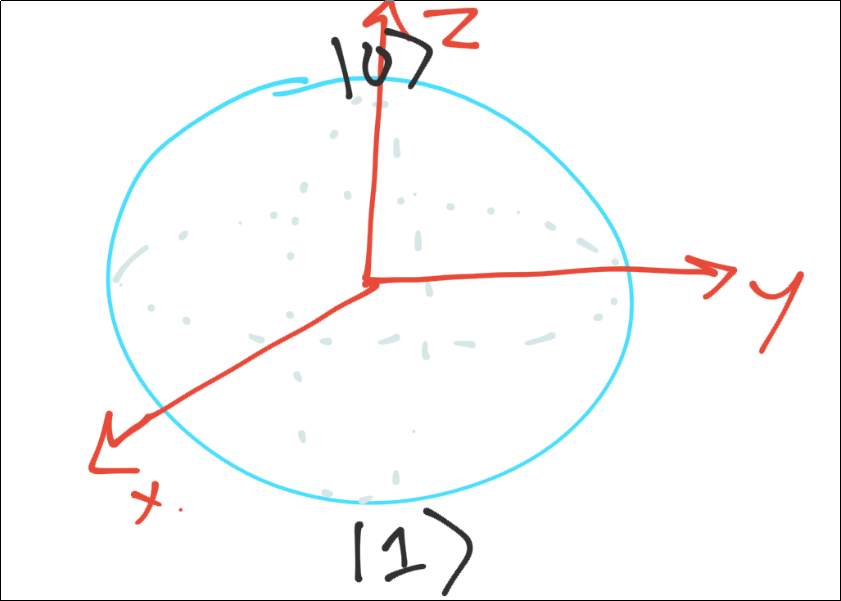At the "top" of the sphere, we have a zero, this is just the notation for the qubit as a zero |0>

The bottom is a 1 |1>

So then our Qubit is a vector in this space. Pretend for me my green line is straight.If we took a measurement, this vector here would collapse to a 0 or a 1. On average, this is going to collapse towards the 0 more often.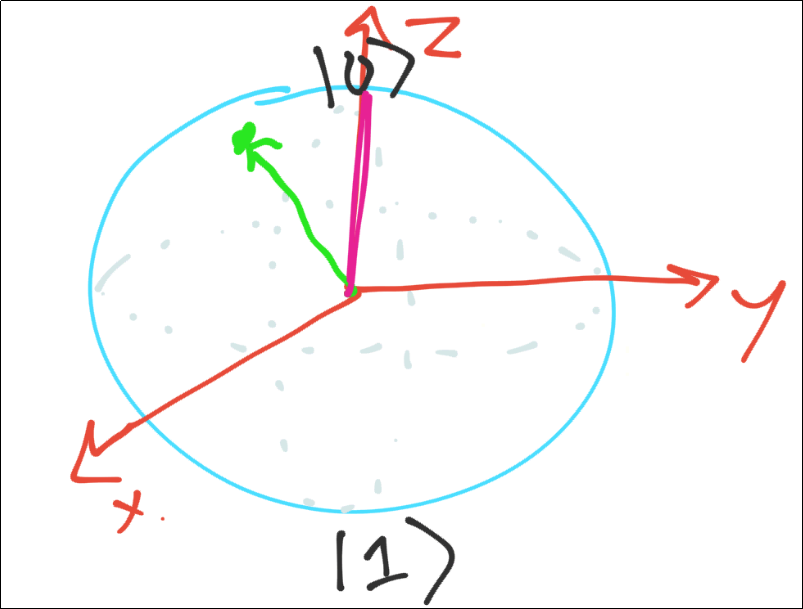The purple line denotes the collapsed vector value after a measurement has been taken. Do note, however, this vector was not perfectly aligned up to the 0. Sometimes, this qubit will be a 1.

If that wasn't enough to deal with, there are more gate operations than just a not (thought even these in succession can do interesting things). If you were to apply a not here, take note what you think would happen.

Next, there are gates that also do other things, like rotating a vector. For example, you might have a gate that takes the following vector: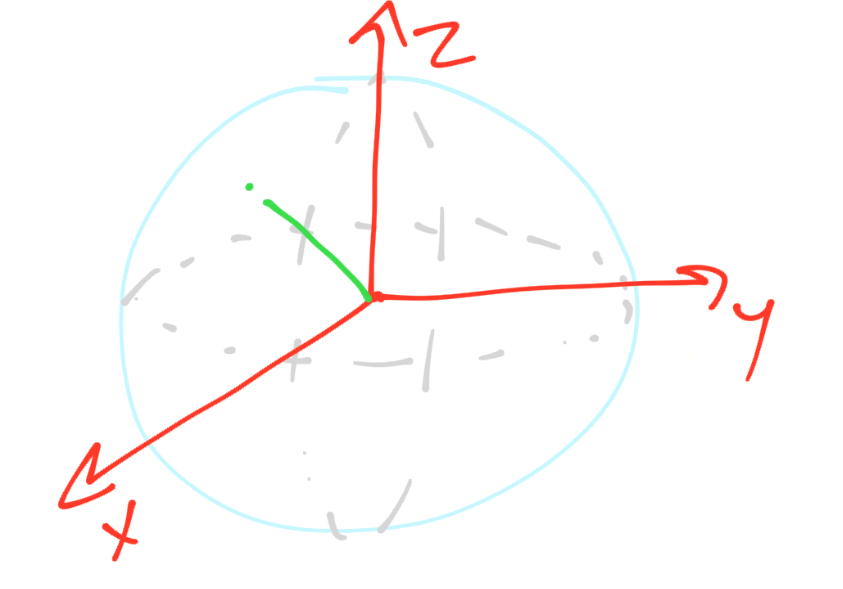and transforms it to: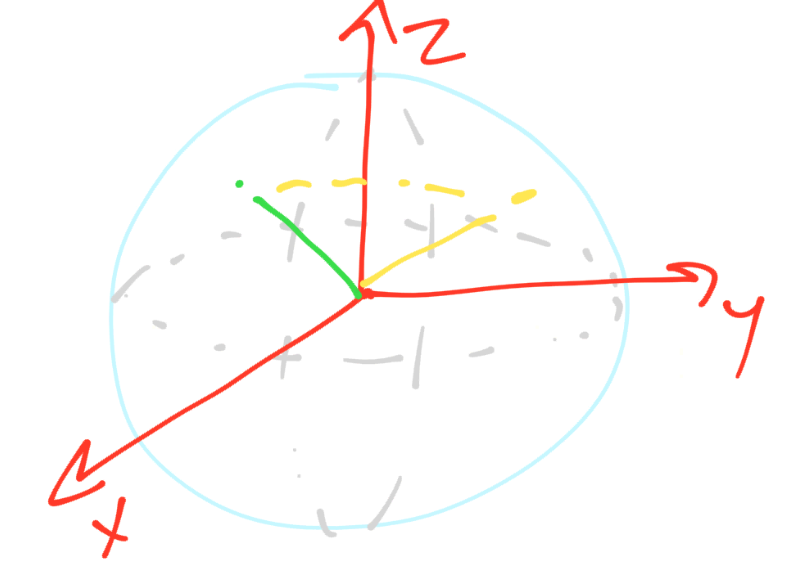Now this might currently collapse with the same probabilities, but there are other gates that might rotate in other ways, other gates that will depend on factors like this.

The best way we can get a feel for this is by tinkering with it, however, so let's tinker...because, to be honest, my drawing skills are not the greatest!

To begin, I am going to make a bunch of imports for things we plan to use:

import qiskit as q
from qiskit.tools.visualization import plot_bloch_multivector
from qiskit.visualization import plot_histogram
from matplotlib import style
#style.use("dark_background") # I am using dark mode notebook, so I use this to see the chart.
# to use dark mode:
# edited '/usr/local/lib/python3.7/dist-packages/qiskit/visualization/bloch.py line 177 self.font_color = 'white'
# edited '/usr/local/lib/python3.7/dist-packages/qiskit/visualization/counts_visualization.py line 206     ax.set_facecolor('#000000')
%matplotlib inline

statevec_simulator = q.Aer.get_backend("statevector_simulator")
qasm_sim = q.Aer.get_backend('qasm_simulator')

def do_job(circuit):
job = q.execute(circuit, backend=statevec_simulator)
result = job.result()
statevec = result.get_statevector()

n_qubits = circuit.n_qubits
circuit.measure([i for i in range(n_qubits)], [i for i in range(n_qubits)])

qasm_job = q.execute(circuit, backend=qasm_sim, shots=1024).result()
counts = qasm_job.get_counts()

return statevec, counts


Okay so now we've got our simulator setup and then we have a function that will run our job for us and return the state vector and the counts for us, since we're going to be doing this a lot. Now we just make a circuit and run it.

circuit = q.QuantumCircuit(2,2)  # 2 qubits, 2 classical bits
statevec, counts = do_job(circuit)


Just an initialized circuit, does nothing. Both qubits should just be 0s always, but let's get a visual:

plot_bloch_multivector(statevec)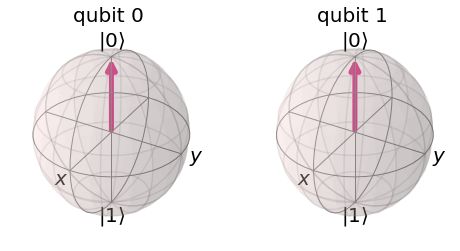So now you can see the vectors! First off, what does adding a Hadamard gate do to the vector? We know this gate puts the qubit in superposition, but what will it look like on the Bloch sphere?

circuit = q.QuantumCircuit(2,2)  # 2 qubits, 2 classical bits
circuit.h(0)  # hadamard gate on qubit0
statevec, counts = do_job(circuit)
plot_bloch_multivector(statevec)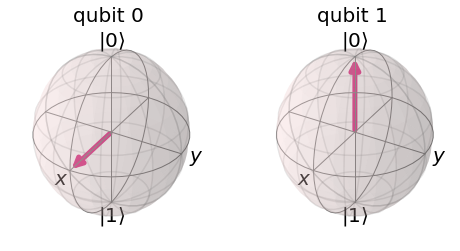Now, what if we entangle qubit 0 and 1 here, with a cnot gate? What will that look like?

circuit = q.QuantumCircuit(2,2)  # 2 qubits, 2 classical bits
circuit.h(0)  # hadamard gate on qubit0
circuit.cx(0,1)  # controlled not control: 0 target: 1
statevec, counts = do_job(circuit)
plot_bloch_multivector(statevec)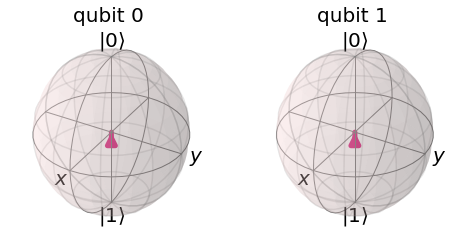Notice how this cnot entanglement, despite having a target on qubit 1, has actually changed qubit 0's vector. From tutorial 1, you might have instead had the impression that a controlled-not gate just impacted the target qubit.

What will the distribution of measurements be here?

plot_histogram([counts], legend=['output'])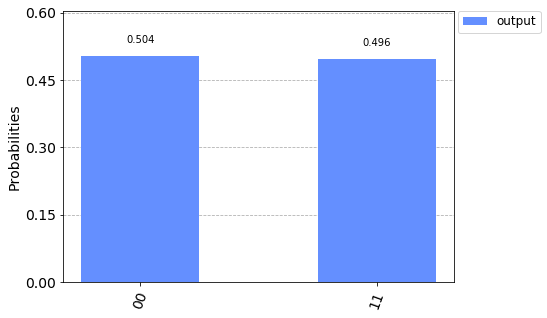No surprise here, I don't think.

What happens if we have 3 qubits, where qubit at index 2 is controlled by qubit 0 and 1, both of which are in superposition? Try to imagine what the Bloch spheres will look like as well as our distribution?

Could we just do:

circuit = q.QuantumCircuit(3,3)  # 2 qubits, 2 classical bits
circuit.h(0)
circuit.h(1)
circuit.cx(0,2)
circuit.cx(1,2)
circuit.draw()

        +---+
q_0: |0>+ H +--#-------
+---+  |
q_1: |0>+ H +--+----#--
+---++-+-++-+-+
q_2: |0>-----+ X ++ X +
+---++---+
c_0: 0 ---------------

c_1: 0 ---------------

c_2: 0 ---------------


It would appear that no, we cannot do this, at least it would not be what we expect. Just to show quickly:

statevec, counts = do_job(circuit)
plot_bloch_multivector(statevec)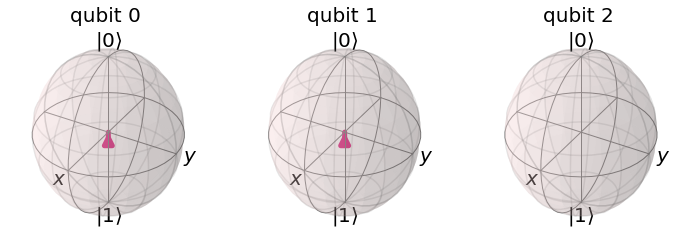plot_histogram([counts], legend=['output'])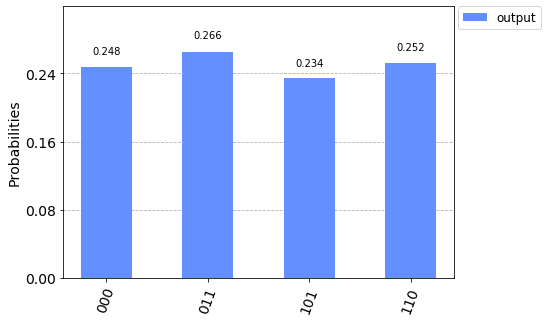Luckily, there is actually a way to do this the way we intended, the ccx gate. This is the controlled controlled not gate! It works like what we intended where the target qubit just has 2 control bits, rather than 1.

Imagine having 2 control qubits, both of which are in superposition and nothing more. What's this mean for your target qubit? At what probability will this qubit be a 1?

circuit = q.QuantumCircuit(3,3)  # 3 qubits, 3 classical bits
circuit.h(0)
circuit.h(1)
circuit.ccx(0,1,2)
circuit.draw()

        +---+
q_0: |0>+ H +--#--
+---+  |
q_1: |0>+ H +--#--
+---++-+-+
q_2: |0>-----+ X +
+---+
c_0: 0 ----------

c_1: 0 ----------

c_2: 0 ----------

statevec, counts = do_job(circuit)
plot_bloch_multivector(statevec)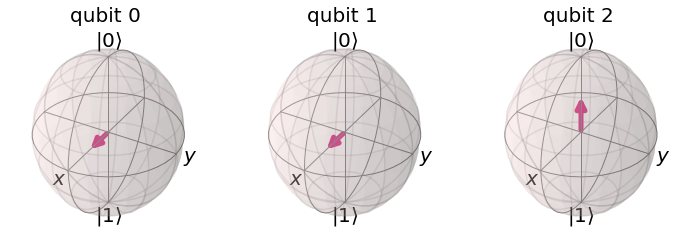One last chance to amend your guesses!

plot_histogram([counts], legend=['output'])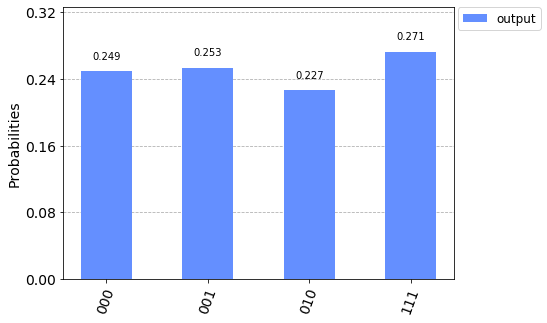So this is a rather interesting distribution, and we could spend some time pondering on it for sure. I am mostly interested in qubit at index 2 though.

Do we always need to have the same size register for our qubits and bits? What about when we go to measure?

When creating our quantum circuit, we absolutely do not need the same sized registers. We might have 500 qubits, but only care about 1 of those qubits, which we'll map to a classical bit.

When we're mapping bits to bits, however, it obviously does matter that we have a 1 to 1 relationship.

So let's take the exact same circuit gates above, but change the circuit slightly:

circuit = q.QuantumCircuit(3,1)  # 3 qubits, only 1 classical bit


So now our circuit will only output 1 classical bit. When we go to measure, we will inform qiskit which qubit will map to this classical bit.

circuit.h(0)  # hadamard
circuit.ccx(0,1,2)  # controlled controlled not
circuit.draw()

        +---+
q_0: |0>+ H +--#--
+---+  |
q_1: |0>+ H +--#--
+---++-+-+
q_2: |0>-----+ X +
+---+
c_0: 0 ----------

circuit.measure(, )  # map qubit @ index 2, to classical bit idx 0.
result = q.execute(circuit, backend=qasm_sim, shots=1024).result()

counts = result.get_counts()
plot_histogram([counts], legend=['output'])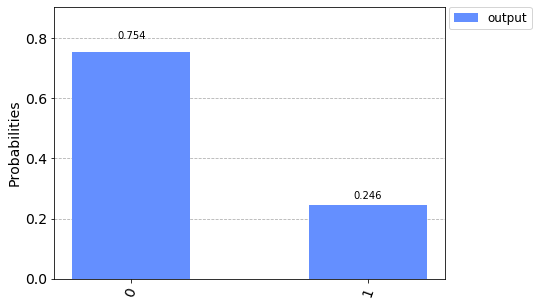Does that make sense to you? Qubit index 2 has a 23% chance of being a 1?

If you have qubit 0 with a 50/50 split of being 0 or 1, and then qubit 1 also has a 50/50 chance. This seems like a 50% chance of a 50% chance, so 25% chance sounds correct to me.

There are also rotations we can apply to a given axis (x, y, z). These rotations may or may not immediately impact the actual measured values immediately, but combinations of gates like this, superpositions, and entanglements compounding these changes can produce interesting results.

import math

circuit = q.QuantumCircuit(3,3)  # even-sized registers again so we can use our function
circuit.ccx(0,1,2)  # controlled controlled not

statevec, counts = do_job(circuit)
plot_bloch_multivector(statevec)So we've seen this one before, nothing too fancy. But, it turns out, there's more to quantum life than controlled nots and superpositions.

How about rotations? We can issue a rotation along any given axis we want. Let's play with the x axis first for qubit @ index 2:

circuit = q.QuantumCircuit(3,3)  # even-sized registers again so we can use our function
circuit.ccx(0,1,2)  # controlled controlled not
circuit.rx(math.pi, 2)

statevec, counts = do_job(circuit)
plot_bloch_multivector(statevec)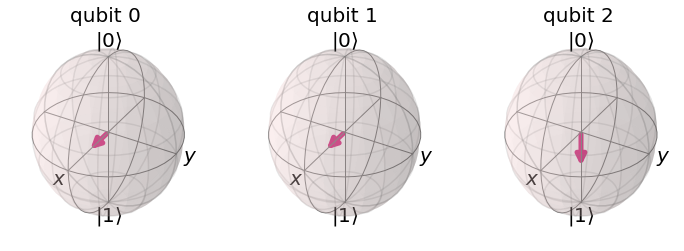This arrow is rotating around that x axis. A half rotation is pi. So pi*2 would put us where we started. What about pi/2?

circuit = q.QuantumCircuit(3,3)  # even-sized registers again so we can use our function
circuit.ccx(0,1,2)  # controlled controlled not
circuit.rx(math.pi/2, 2)

statevec, counts = do_job(circuit)
plot_bloch_multivector(statevec)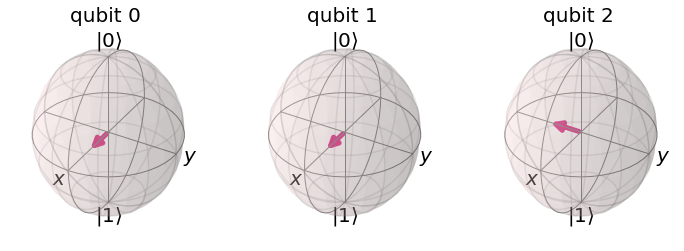Oh, that's interesting. Looks like we've found ourself a way to entangle this qubit with 2 others, each in a 50/50 state...and yet... is this also not in a 25% state, but actually in a 50/50 state too? Let's see:

circuit = q.QuantumCircuit(3,3)
circuit.ccx(0,1,2)  # controlled controlled not
circuit.rx(math.pi/2, 2)
circuit.draw()

        +---+
q_0: |0>+ H +--#--------------
+---+  |
q_1: |0>+ H +--#--------------
+---++-+-++----------+
q_2: |0>-----+ X ++ Rx(pi/2) +
+---++----------+
c_0: 0 ----------------------

c_1: 0 ----------------------

c_2: 0 ----------------------

circuit.measure(, )  # map qubit @ index 2, to classical bit idx 0.
result = q.execute(circuit, backend=qasm_sim, shots=1024).result()
counts = result.get_counts()
plot_histogram([counts], legend=['output'])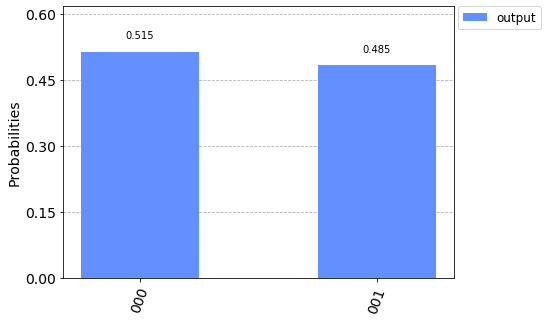It sure is back in the 50/50 state, despite being controlled by a 50% chance * another 50% chance. Very interesting! So we're able to use our circuits to create intersting versions of these relationships. Could it get any more interesting? So far we've been working with making our Qubits whole numbers on specific axis and it's been easy for us to presume what's going to happen.

circuit = q.QuantumCircuit(3,3)  # even-sized registers again so we can use our function